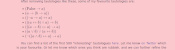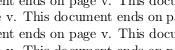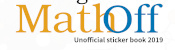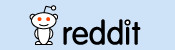mscroggs.co.uk
mscroggs.co.uksubscribe

# Blog

2019-03-26
I originally wrote this post for The Aperiodical.
A few months ago, Adam Townsend went to lunch and had a conversation. I wasn't there, but I imagine the conversation went something like this:Smitha: Hello.
Smitha: You know how the \hat command in LaTeΧ puts a caret above a letter?... Well I was thinking it would be funny if someone made a package that made the \hat command put a picture of an actual hat on the symbol instead?
Adam: (After a few hours of laughter.) I'll see what my flatmate is up to this weekend...
Jeff: What on Earth are you two talking about?!As anyone who has been anywhere near maths at a university in the last ∞ years will be able to tell you, LaTeΧ is a piece of maths typesetting software. It's a bit like a version of Word that runs in terminal and makes PDFs with really pretty equations.
By default, LaTeΧ can't do very much, but features can easily added by importing packages: importing the graphicsx package allows you to put images in your PDF; importing geometry allows you to easily change the page margins; and importing realhats makes the \hat command put real hats above symbols.

### Changing the behaviour of \hat

By default, the LaTeΧ command \hat puts a pointy "hat" above a symbol:
After Adam's conversation, we had a go at redefining the \hat command by putting the following at the top of our LaTeΧ file.
LaTeΧ
\renewcommand{\hat}{
% We put our new definition here
}

After a fair amount of fiddling with the code, we eventually got it to produce the following result:a (left) and \hat{a} (right) while using the realhats package
We were now ready to put our code into a package so others could use it.

### How to write a package

A LaTeΧ package is made up of:
• a sty file, containing a collection of commands like the one we wrote above;
• a PDF of documentation showing users how to use your package;
It's quite common to make the first two of these by making a dtx file and an ins file. And no, we have no idea either why these are the file extensions used or why this is supposedly simpler than making a sty file and a PDF.
The ins file says which bits of the dtx should be used to make up the sty file. Our ins file looks like this:
LaTeΧ
\input{docstrip.tex}
\keepsilent
\usedir{tex/latex/realhats}
\preamble
\endpreamble
\generate{
\file{realhats.sty}{\from{realhats.dtx}{realhats}}
}
\endbatchfile
The most important command in this file is \generate: this says that that the file realhats.sty should be made from the file realhats.dtx taking all the lines that are marked as part of realhats. The following is part of our dtx file:
LaTeΧ
%\lstinline{realhats} is a package for \LaTeX{} that makes the \lstinline{\hat}
%command put real hats on symbols.
%For example, the input \lstinline@\hat{a}=\hat{b}@ will produce the output:
%$\hat{a}=\hat{b}$
%To make a vector with a hat, the input \lstinline@\hat{\mathbf{a}}@ produces:
%$\hat{\mathbf{a}}$
%
%\iffalse
%<*documentation>
\documentclass{article}
\usepackage{realhats}
\usepackage{doc}
\usepackage{listings}
\title{realhats}
\begin{document}
\maketitle
\DocInput{realhats.dtx}
\end{document}
%</documentation>
%\fi

%\iffalse
%<*realhats>
\NeedsTeXFormat{LaTeX2e}
\ProvidesPackage{realhats}[2019/02/02 realhats]

\RequirePackage{amsmath}
\RequirePackage{graphicx}
\RequirePackage{ifthen}
\renewcommand{\hat}{
% We put our new definition here
}
%</realhats>
%\fi
The lines near the end between <*realhats> and </realhats> will be included in the sty file, as they are marked at part of realhats.
The rest of this file will make the PDF documentation when the dtx file is compiled. The command \DocInput tells LaTeΧ to include the dtx again, but with the %s that make lines into comments removed. In this way all the comments that describe the functionality will end up in the PDF. The lines that define the package will not be included in the PDF as they are between \iffalse and \fi.
Writing both the commands and the documentation in the same file like this means that the resulting file is quite a mess, and really quite ugly. But this is apparently the standard way of writing LaTeΧ packages, so rest assured that it's not just our code that ugly and confusing.

### What to do with your package

Once you've written a package, you'll want to get it out there for other people to use. After all, what's the point of being able to put real hats on top of symbols if the whole world can't do the same?
First, we put the source code of our package on GitHub, so that Adam and I had an easy way to both work on the same code. This also allows other LaTeΧ lovers to see the source and contribute to it, although none have chosen to add anything yet.
Next, we submitted our package to CTAN, the Comprehensive TeΧ Archive Network. CTAN is an archive of thousands of LaTeΧ packages, and putting realhats there gives LaTeΧ users everywhere easy access to real hats. Within days of being added to CTAN, realhats was added (with no work by us) to MikTeX and TeX Live to allow anyone using these LaTeΧ distributions to seemlessly install it as soon as it is needed.
We figured that the packaged needed a website too, so we made one. We also figured that the website should look as horrid as possible.

### How to use realhats

So if you want to end fake hats and put real hats on top of your symbols, you can simply write \usepackage{realhats} at the top of your LaTeΧ file.realhats: gotta put them all in academic papers

### Similar postsA surprising fact about quadrilateralsInteresting tautologiesA non-converging LaTeX documentBig Internet Math-Off stickers 2019

Comments in green were written by me. Comments in blue were not written by me.

Allowed HTML tags: <br> <a> <small> <b> <i> <s> <sup> <sub> <u> <spoiler> <ul> <ol> <li>
To prove you are not a spam bot, please type "x-axis" in the box below (case sensitive):
2015-08-29
A few weeks ago, I made OEISbot, a Reddit bot which posts information whenever an OEIS sequence is mentioned.
This post explains how OEISbot works. The full code can be found on GitHub.

### Getting started

OEISbot is made in Python using PRAW (Python Reddit Api Wrapper). PRAW can be installed with:
bash
pip install praw
Before making a bot, you will need to make a Reddit account for your bot, create a Reddit app and obtain API keys. This python script can be used to obtain the necessary keys.
Once you have your API keys saved in your praw.ini file, you are ready to make a bot.

### Writing the bot

First, the necessary imports are made, and test mode is activated if the script is run with test as an argument. We also define an exception that will be used later to kill the script once it makes a comment.
python
import praw
import re
import urllib
import json

import sys
test = False
if len(sys.argv) > 1 and sys.argv == "test":
test = True
print("TEST MODE")

class FoundOne(BaseException):
pass

To prevent OEISbot from posting multiple links to the same sequence in a thread, lists of sequences linked to in each thread can be loaded and saved using the following functions.
python
def save_list(seen, _id):
print(seen)
with open("/home/pi/OEISbot/seen/"+_id, "w"as f:
return json.dump(seen, f)

def open_list(_id):
try:
with open("/home/pi/OEISbot/seen/" + _id) as f:
except:
return []
The following function will search a post for a mention of an OEIS sequence number.
python
def look_for_A(id_, text, url, comment):
seen = open_list(id_)
re_s = re.findall("A([0-9]{6})", text)
re_s += re.findall("oeis\.org/A([0-9]{6})", url)
if test:
print(re_s)
post_me = []
for seq_n in re_s:
if seq_n not in seen:
post_me.append(markup(seq_n))
seen.append(seq_n)
if len(post_me) > 0:
post_me.append(me())
comment(joiner().join(post_me))
save_list(seen, id_)
raise FoundOne
The following function will search a post for a comma-separated list of numbers, then search for it on the OEIS. If there are 14 sequences or less found, it will reply. If it finds a list with no matches on the OEIS, it will message /u/PeteOK, as he likes hearing about possibly new sequences.
python
def look_for_ls(id_, text, comment, link, message):
seen = open_list(id_)
if test:
print(text)
re_s = re.findall("([0-9]+\, *(?:[0-9]+\, *)+[0-9]+)", text)
if len(re_s) > 0:
for terms in ["".join(i.split(" ")) for i in re_s]:
if test:
print(terms)
if terms not in seen:
seen.append(terms)
if test:
print(first10)
if len(first10)>and total <= 14:
if total == 1:
intro = "Your sequence (" + terms \
+ ") looks like the following OEIS sequence."
else:
intro = "Your sequence (" + terms + \
+ ") may be one of the following OEIS sequences."
if total > 4:
intro += " Or, it may be one of the " + str(total-4) \
+ " other sequences listed [here]" \
"(http://oeis.org/search?q=" + terms + ")."
post_me = [intro]
if test:
print(first10)
for seq_n in first10[:4]:
post_me.append(markup(seq_n))
seen.append(seq_n)
post_me.append(me())
comment(joiner().join(post_me))
save_list(seen, id_)
raise FoundOne
elif len(first10) == 0:
post_me = ["I couldn't find your sequence (" + terms \
+ ") in the [OEIS](http://oeis.org). "
message("PeteOK",
"Sequence not in OEIS",
"Hi Peter, I've just found a new sequence (" \
"Please shout at /u/mscroggs to turn the " \
"feature off if its spamming you!")
post_me.append(me())
comment(joiner().join(post_me))
save_list(seen, id_)
raise FoundOne

ls = re.findall("href=(?:'|\")/A([0-9]{6})(?:'|\")", src)
try:
tot = int(re.findall("of ([0-9]+) results found", src))
except:
tot = 0
return ls, tot
The markup function loads the necessary information from OEIS and formats it. Each comment will end with the output of the me function. The ouput of joiner will be used between sequences which are mentioned.
python
def markup(seq_n):
pattern = re.compile("%N (.*?)<", re.DOTALL|re.M)
desc = urllib.urlopen("http://oeis.org/A" + seq_n + "/internal").read()
desc = pattern.findall(desc).strip("\n")
pattern = re.compile("%S (.*?)<", re.DOTALL|re.M)
seq = urllib.urlopen("http://oeis.org/A" + seq_n + "/internal").read()
seq = pattern.findall(seq).strip("\n")
new_com = "[A" + seq_n + "](http://oeis.org/A" + seq_n + "/): "
new_com += desc + "\n\n"
new_com += seq + "..."
return new_com

def me():
return "I am OEISbot. I was programmed by /u/mscroggs. " \
"[How I work](http://mscroggs.co.uk/blog/20). " \
"You can test me and suggest new features at /r/TestingOEISbot/."

def joiner():
return "\n\n- - - -\n\n"
Next, OEISbot logs into Reddit.
python
= praw.Reddit("OEIS link and description poster by /u/mscroggs.")

access_i = r.refresh_access_information(refresh_token=r.refresh_token)
r.set_access_credentials(**access_i)

auth = r.get_me()
The subs which OEISbot will search through are listed. I have used all the math(s) subs which I know about, as these will be the ones mentioning sequences.
python
subs = ["TestingOEISbot","math","mathpuzzles","casualmath","theydidthemath",
"learnmath","mathbooks","cheatatmathhomework","matheducation",
"recreationalmath","OEIS","mathclubs","maths"]
if test:
subs = ["TestingOEISbot"]
For each sub OEISbot is monitoring, the hottest 10 posts are searched through for mentions of sequences. If a mention is found, a reply is generated and posted, then the FoundOne exception will be raised to end the code.
python
try:
for sub in subs:
print(sub)
subreddit = r.get_subreddit(sub)
for submission in subreddit.get_hot(limit = 10):
if test:
print(submission.title)
look_for_A(submission.id,
submission.title + "|" + submission.selftext,
submission.url,
look_for_ls(submission.id,
submission.title + "|" + submission.selftext,
submission.url,
r.send_message)

and comment.author is not None
and comment.author.name != "OEISbot" ):
look_for_A(submission.id,
re.sub("$[^$]*\]$$[^$$*]\)","",comment.body),
comment.body,
look_for_ls(submission.id,
re.sub("$[^$]*\]$$[^$$*]\)","",comment.body),
submission.url,
r.send_message)

except FoundOne:
pass

### Running the code

I put this script on a Raspberry Pi which runs it every 10 minutes (to prevent OEISbot from getting refusals for posting too often). This is achieved with a cron job.
bash
*/10 * * * * python /path/to/bot.py

The full OEISbot code is available on GitHub. Feel free to use it as a starting point to make your own bot! If your bot is successful, let me know about it in the comments below or on Twitter.

### Similar postsLogic botRaspberry Pi weather stationLogical contradictionsLogic bot, pt. 2

Comments in green were written by me. Comments in blue were not written by me.

Allowed HTML tags: <br> <a> <small> <b> <i> <s> <sup> <sub> <u> <spoiler> <ul> <ol> <li>
To prove you are not a spam bot, please type "l" then "i" then "n" then "e" then "a" then "r" in the box below (case sensitive):
2014-11-26
Last week, mathslogicbot started the long task of tweeting every tautology in propositional calculus. This post explains what this means and how I did it.

### What is propositional calculus?

Propositional calculus is a form of mathematical logic, in which the formulae (the logical 'sentences') are made up of the following symbols:
• Variables (a to z and $$\alpha$$ to $$\lambda$$) (Variables are usually written as $$p_1$$, $$p_2$$, etc. but as Twitter cannot display subscripts, I chose to use letters instead.)
• Not ($$\neg$$)
• Implies ($$\rightarrow$$)
• If and only if ($$\leftrightarrow$$)
• And ($$\wedge$$)
• Or ($$\vee$$)
• Brackets ($$()$$)

#### Formulae

Formulae are defined recursively using the following rules:
• Every variable is a formula.
• If $$A$$ is a formula, then $$\neg A$$ is a formula.
• If $$A$$ and $$B$$ are formulae then $$(A\rightarrow B)$$, $$(A\leftrightarrow B)$$, $$(A\wedge B)$$ and $$(A\vee B)$$ are all formulae.
For example, $$(a\vee b)$$, $$\neg f$$ and $$((a\vee b)\rightarrow\neg f)$$ are formulae.
Each of the variables is assigned a value of either "true" or "false", which leads to each formula being either true or false:
• $$\neg a$$ is true if $$a$$ is false (and false otherwise).
• $$(a\wedge b)$$ is true if $$a$$ and $$b$$ are both true (and false otherwise).
• $$(a\vee b)$$ is true if $$a$$ or $$b$$ is true (or both are true) (and false otherwise).
• $$(a\leftrightarrow b)$$ is true if $$a$$ and $$b$$ are either both true or both false (and false otherwise).
• ($$a \rightarrow\ b)$$ is true if $$a$$ and $$b$$ are both true or $$a$$ is false (and false otherwise).

#### Tautologies

A tautology is a formula that is true for any assigment of truth values to the variables. For example:
$$(a\vee \neg a)$$ is a tautology because: if $$a$$ is true then $$a$$ or $$\neg a$$ is true; and if $$a$$ is false, then $$\neg a$$ is true, so $$a$$ or $$\neg a$$ is true.
$$(a\leftrightarrow a)$$ is a tautology because: if $$a$$ is true then $$a$$ and $$a$$ are both true; and if $$a$$ is false then $$a$$ and $$a$$ are both false.
$$(a\wedge b)$$ is not a tautology because if $$a$$ is true and $$b$$ is false, then it is false.
The following are a few more tautologies. Can you explain why they are always true?
• $$(a\leftrightarrow a)$$
• $$((a\vee\neg a)\vee a)$$
• $$\neg(a\wedge\neg a)$$
• $$(a\vee(a\rightarrow b))$$

### Python

If you want to play with the Logic Bot code, you can download it here.
In order to find all tautologies less than 140 characters long, one method is to first generate all formulae less than 140 characters then check to see if they are tautologies. (This is almost certainly not the fastest way to do this, but as long as it generates tautolgies faster than I want to tweet them, it doesn't matter how fast it runs.) I am doing this on a Raspberry Pi using Python in the following way.

#### All formulae

The following code is writing all the formulae that are less than 140 characters to a file called formulae.
python
from os.path import join
path = "/home/pi/logic"
First import any modules needed and set the path where the file will be saved.
python
def candidate(formula):
global formulae

if len(formula) <= 140 and formula not in formulae:
formulae.append(formula)
print formula
f = open(join(path,"formulae"),"a")
f.write(formula + "\n")
f.close()
This function checks that a formula is not already in my list of formulae and shorter than 140 characters, then adds it to the list and writes it into the file.
python
variables = ["a""b""c""d""e""f""g""h""i""j",
"k""l""m""n""o""p""q""r""s""t",
"u""v""w""x""y""z""@""#""2""3",
"4""5""6""7""8""9"]
This line says which characters are going to be used as variables. It is impossible to write a formula in less that 140 characters with more than 36 different variables so these will be sufficient. I haven't used 0 and 1 as these are used to represent false and true later.
python
= open(join(path,"formulae"))
for i in range(0,len(formulae)):
formulae[i] = formulae[i].strip("\n")
f.close()
These lines load the formulae already found from the file. This is needed if I have to stop the code then want to continue.
python
oldlen = 0
newlen = 26

while oldlen != newlen:
for f in formulae + variables:
candidate("-" + f)
for f in formulae + variables:
for g in formulae:
for star in ["I""F""N""U"]:
candidate("(" + f + star + g + ")")
oldlen = newlen
newlen = len(formulae)
The code inside the while loop goes through every formula already found and puts "-" in front of it, then takes every pair of formulae already found and puts "I", "F", "N" or "U" between them. These characters are used instead of the logical symbols as using the unicode characters leads to numerous python errors. The candidate function as defined above then adds them to the list (if they are suitable). This continues until the loop does not make the list of formulae longer as this will occur when all formulae are found.
python
= open(join(path,"formulae"),"a")
f.write("#FINISHED#")
f.close()
Once the loop has finished this will add the string "#FINISHED#" to the file. This will tell the truth-checking code when the it has checked all the formulae (opposed to having checked all those generated so far).

#### Tautologies

Now that the above code is finding all formulae, I need to test which of these are tautologies. This can be done by checking whether every assignment of truth values to the variables will lead to the statement being true.
python
from os.path import join
path = "/home/pi/logic"
First import any modules needed and set the path where the file will be saved.
python
def next(ar,i=0):
global cont
if i < len(ar):
if ar[i] == "0":
ar[i] = "1"
else:
ar[i] = "0"
ar = next(ar, i + 1)
else:
cont = False
return ar
Given an assignment of truth values, this function will return the next assignment, setting cont to False if all the assignments have been tried.
python
def solve(lo):
lo = lo.replace("-0""1")
lo = lo.replace("-1""0")

lo = lo.replace("(0I0)""1")
lo = lo.replace("(0I1)""1")
lo = lo.replace("(1I0)""0")
lo = lo.replace("(1I1)""1")

lo = lo.replace("(0F0)""1")
lo = lo.replace("(0F1)""0")
lo = lo.replace("(1F0)""0")
lo = lo.replace("(1F1)""1")

lo = lo.replace("(0N0)""0")
lo = lo.replace("(0N1)""0")
lo = lo.replace("(1N0)""0")
lo = lo.replace("(1N1)""1")

lo = lo.replace("(0U0)""0")
lo = lo.replace("(0U1)""1")
lo = lo.replace("(1U0)""1")
lo = lo.replace("(1U1)""1")

return lo
This function will replace all instances of "NOT TRUE" with "FALSE" and so on. It will be called repeatedly until a formula is reduced to true or false.
python
= open(join(path,"formulae"))
f.close()

= open(join(path,"donet"))
f.close()

variables = ["a""b""c""d""e""f""g""h""i""j",
"k""l""m""n""o""p""q""r""s""t",
"u""v""w""x""y""z""@""#""2""3",
"4""5""6""7""8""9"]
These lines read the formulae from the file they are saved in and load how many have been checked if this script has been restarted. The the variables are set.
python
while formulae[-1] != "#FINISHED#" or i < len(formulae) - 1:
if i < len(formulae):
formula = formulae[i].strip("\n")
These lines will loop through all formulae until "#FINISHED#" is reached.
python
insofar = True
inA = []
fail = False
for a in variables:
if a not in formula:
insofar = False
elif not insofar:
fail = True
break
else:
inA.append(a)
Here, the code checks that if a variable is in the formula, then all the previous variables are in the formula. This will prevent the Twitter bot from repeating many tautologies that are the same except for the variable a being replaced by b (although there will still be some repeats like this. Can you work out what these will be?).
python
if not fail:
valA = ["0"]*len(inA)
cont = True
taut = True
while cont and taut:
feval = formula
for j in range(0,len(inA)):
feval = feval.replace(inA[j],valA[j])
while feval not in ["0""1"]:
feval = solve(feval)
if feval != "1":
taut = False
valA = next(valA)
if taut:
f = open(join(path,"true"),"a")
f.write(str(formula) + "\n")
f.close()

i += 1
f = open(join(path,"donet"),"w")
f.write(str(i))
f.close()
Now, the formula is tested to see if it is true for every assignment of truth values. If it is, it is added to the file containing tautologies. Then the number of formulae that have been checked is written to a file (in case the script is stopped then resumed).
python
else:
f = open(join(path,"formulae"))
f.close()
If the end of the formulae file is reached, then the file is re-loaded to include all the formulae found while this code was running.

#### Tweeting

Finally, I wrote a code that tweets the next item in the file full of tautologies every three hours (after replacing the characters with the correct unicode characters).

### How long will it take?

Now that the bot is running, it is natural to ask how long it will take to tweet all the tautologies.
While it is possible to calculate the number of formulae with 140 characters or less, there is no way to predict how many of these will be tautologies without checking. However, the bot currently has over 13 years of tweets lines up. And all the tautologies so far are under 30 characters so there are a lot more to come...
Edit: Updated time left to tweet.

### Similar postsLogical contradictionsLogic bot, pt. 2How OEISbot worksRaspberry Pi weather station

Comments in green were written by me. Comments in blue were not written by me.
In part two you say a_{n+4} >= 2*a_n, and you have 13 years worth of tweets of length (say) 15-30. so there are 26 years worth length 19-34 characters, 13*2^n years worth of tweets of length between (15 + 4n) and (30 + 4n). In particular, setting n = 27, we have 13*2^{27} = 1744830464 years worth of tweets of length 123-138. I hope you have nice sturdy hardware!

Christian

Allowed HTML tags: <br> <a> <small> <b> <i> <s> <sup> <sub> <u> <spoiler> <ul> <ol> <li>
To prove you are not a spam bot, please type "theorem" in the box below (case sensitive):
2013-07-11
On 19 June, the USB temperature sensor I ordered from Amazon arrived. This sensor is now hooked up to my Raspberry Pi, which is taking the temperature every 10 minutes, drawing graphs, then uploading them here. Here is a brief outline of how I set this up:

I found this code and adapted it to write the date, time and temperature to a text file. I then set cron to run this every 10 minutes. It writes the data to a text file (/var/www/temperature2) in this format:
2013 06 20 03 50,16.445019
2013 06 20 04 00,16.187843
2013 06 20 04 10,16.187843
2013 06 20 04 20,16.187843

### Plotting the graphs

I found a guide somewhere on the internet about how to draw graphs with Python using Pylab/Matplotlib. If you have any idea where this could be, comment below and I'll put a link here.
In the end my code looked like this:
python
import time
import matplotlib as mpl
mpl.use("Agg")
import matplotlib.pylab as plt
import matplotlib.dates as mdates

ts = time.time()
import datetime
now = datetime.datetime.fromtimestamp(ts)
st = mdates.date2num(datetime.datetime(int(float(now.strftime("%Y"))),
int(float(now.strftime("%m"))),
int(float(now.strftime("%d"))),
0, 0, 0))
weekst = st - int(float(datetime.datetime.fromtimestamp(ts).strftime("%w")))
= file("/var/www/temperature2","r")
= []
= []
tt = []
ss = []
= []
= []
= []
= []
= []
weekt = []
weektt = []
weeks = []
weekss = []
mini = 1000
maxi = 0
cur = -1
datC = 0

for line in f:
fL = line.split(",")
fL = fL.split(" ")
if cur == -1:
cur = mdates.date2num(datetime.datetime(int(float(fL)),
int(float(fL)),
int(float(fL)),
0,0,0))
datC = mdates.date2num(datetime.datetime(int(float(fL)),
int(float(fL)),
int(float(fL)),
int(float(fL)),
int(float(fL)),
0))
u.append(datC)
v.append(fL)
if datC >= st and datC <= st + 1:
t.append(datC)
s.append(fL)
if datC >= st - 1 and datC <= st:
tt.append(datC + 1)
ss.append(fL)
if datC >= weekst and datC <= weekst + 7:
weekt.append(datC)
weeks.append(fL)
if datC >= weekst - 7 and datC <= weekst:
weektt.append(datC + 7)
weekss.append(fL)
if datC > cur + 1:
g.append(cur)
h.append(mini)
i.append(maxi)
mini = 1000
maxi = 0
cur = mdates.date2num(datetime.datetime(int(float(fL)),
int(float(fL)),
int(float(fL)),
0,0,0))
mini = min(float(fL),mini)
maxi = max(float(fL),maxi)
g.append(cur)
h.append(mini)
i.append(maxi)

plt.plot_date(x=t,y=s,fmt="r-")
plt.plot_date(x=tt,y=ss,fmt="g-")
plt.xlabel("Time")
plt.ylabel("Temperature (#^\circ#C)")
plt.title("Daily")
plt.legend(["today","yesterday"], loc="upper left",prop={"size":8})
plt.grid(True)
plt.gca().xaxis.set_major_formatter(mdates.DateFormatter("%H:%M"))
labels = plt.gca().get_xticklabels()
plt.setp(labels,rotation=90,fontsize=10)
plt.xlim(st,st + 1)
plt.savefig("/var/www/tempr/tg1p.png")

plt.clf()

plt.plot_date(x=weekt,y=weeks,fmt="r-")
plt.plot_date(x=weektt,y=weekss,fmt="g-")
plt.xlabel("Day")
plt.ylabel("Temperature (#^\circ#C)")
plt.title("Weekly")
plt.legend(["this week","last week"], loc="upper left",prop={"size":8})
plt.grid(True)
plt.gca().xaxis.set_major_formatter(mdates.DateFormatter("                     %A"))
labels = plt.gca().get_xticklabels()
plt.setp(labels,rotation=0,fontsize=10)
plt.xlim(weekst,weekst + 7)
plt.savefig("/var/www/tempr/tg2p.png")

plt.clf()

plt.plot_date(x=u,y=v,fmt="r-")
plt.xlabel("Date & Time")
plt.ylabel("Temperature (#^\circ#C)")
plt.title("Forever")
plt.grid(True)
plt.gca().xaxis.set_major_formatter(mdates.DateFormatter("%d/%m/%y %H:%M"))
labels = plt.gca().get_xticklabels()
plt.setp(labels,rotation=90,fontsize=8)
plt.savefig("/var/www/tempr/tg4p.png")

plt.clf()

plt.plot_date(x=g,y=h,fmt="b-")
plt.plot_date(x=g,y=i,fmt="r-")
plt.xlabel("Date")
plt.ylabel("Temperature (#^\circ#C)")
plt.title("Forever")
plt.legend(["minimum","maximum"], loc="upper left",prop={"size":8})
plt.grid(True)
plt.gca().xaxis.set_major_formatter(mdates.DateFormatter("%d %b"))
labels = plt.gca().get_xticklabels()
plt.setp(labels,rotation=90,fontsize=8)
plt.savefig("/var/www/tempr/tg3p.png")
If there's anything in there you don't understand, comment below and I'll try to fill in the gaps.

Finally, I upload the graphs to mscroggs.co.uk/weather. To do this, I set up pre-shared keys on the Raspberry Pi and this server and added the following as a cron job:
bash
0 * * * * scp /var/www/tempr/tg*p.png username@mscroggs.co.uk:/path/to/folder
I hope this was vaguely interesting/useful. I'll try to add more details and updates over time. If you are building something similar, please let me know in the comments; I'd love to see what everyone else is up to.
Edit: Updated to reflect graphs now appearing on mscroggs.co.uk not catsindrag.co.uk.

### Similar postsHow OEISbot worksLogic botLogical contradictionsLogic bot, pt. 2

Comments in green were written by me. Comments in blue were not written by me.